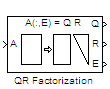# QR Factorization

Factor arbitrary matrix into unitary and upper triangular components

## Library

Math Functions / Matrices and Linear Algebra / Matrix Factorizations

`dspfactors`

•## Description

The QR Factorization block uses a sequence of Householder transformations to triangularize the input matrix A. The block factors a column permutation of the M-by-N input matrix A as

Ae = QR

The column-pivoted matrix Ae contains the columns of A permuted as indicated by the contents of length-N permutation vector E.

```Ae = A(:,E) % Equivalent MATLAB code ```

The block selects a column permutation vector E, which ensures that the diagonal elements of matrix R are arranged in order of decreasing magnitude.

The size of matrices Q and R depends on the setting of the Output size parameter:

• When you select `Economy` for the output size, Q is an M-by-min(M,N) unitary matrix, and R is a min(M,N)-by-N upper-triangular matrix.

`[Q R E] = qr(A,0) % Equivalent MATLAB code`

• When you select `Full` for the output size, Q is an M-by-M unitary matrix, and R is a M-by-N upper-triangular matrix.

`[Q R E] = qr(A) % Equivalent MATLAB code`

The block treats length-M unoriented vector input as an M-by-1 matrix.

QR factorization is an important tool for solving linear systems of equations because of good error propagation properties and the invertibility of unitary matrices:

Q –1 = Q`'`

where Q`'` is the complex conjugate transpose of Q.

Unlike LU and Cholesky factorizations, the matrix A does not need to be square for QR factorization. However, QR factorization requires twice as many operations as LU Factorization (Gaussian elimination).

## Examples

The Output size parameter of the QR factorization block has two settings: `Economy` and `Full`. When the M-by-N input matrix A has dimensions such that M > N, the dimensions of output matrices Q and R differ depending on the setting of the Output size parameter. If, however, the size of the input matrix A is such that MN, output matrices Q and R have the same dimensions, regardless of whether the Output size is set to `Economy` or `Full`.

The input to the QR Factorization block in the following model is a `5`-by-`2` matrix A. When you change the setting of the Output size parameter from `Economy` to `Full`, the dimensions of the output given by the QR Factorization block also change.

1. Open the model by typing `ex_qrfactorization_ref` at the MATLAB® command line.

2. Double-click the QR Factorization block, set the Output size parameter to `Economy`, and run the model.The QR Factorization block outputs a `5`-by-`2` matrix Q and a `2`-by-`2` matrix R.

3. Change the Output size parameter of the QR Factorization block to `Full` and rerun the model.The QR Factorization block outputs a `5`-by-`5` matrix Q and a `5`-by-`2` matrix R.

## Parameters

Output size

Specify the size of output matrices Q and R:

• `Economy` — When this output size is selected, the block outputs an M-by-min(M,N) unitary matrix Q and a min(M,N)-by-N upper-triangular matrix R.

• `Full` — When this output size is selected, the block outputs an M-by-M unitary matrix Q and a M-by-N upper-triangular matrix R.

Simulate using

• `Interpreted execution` (default)

Simulate model using the MATLAB interpreter. This option shortens startup time and has faster simulation speed compared to `Code generation`.

• `Code generation`

Simulate model using generated C code. The first time you run a simulation, Simulink® generates C code for the block. The C code is reused for subsequent simulations, as long as the model does not change. This option requires additional startup time but provides faster subsequent simulations.

## References

Golub, G. H., and C. F. Van Loan. Matrix Computations. 3rd ed. Baltimore, MD: Johns Hopkins University Press, 1996.

## Supported Data Types

PortSupported Data Types

Input

• Double-precision floating point

• Single-precision floating point

Output

• Double-precision floating point

• Single-precision floating point

## Extended Capabilities

### Topics

Introduced before R2006a

## SupportGet trial now